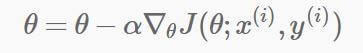#### 用SGD计算逻辑回归时的迭代公式是什么？

统计/机器学习 回归分析 监督式学习 损失函数    浏览次数：1909        分享

0

SGD的公式如下，alpha后面的是在xi, yi点的损失函数的梯度huanx8t   2018-07-27 17:20

##### 2个回答
4

$$\theta_j:=\theta_j - \alpha (h_\theta(x^r) - y^r)x^r_j$$

$h_\theta(x^r)$是当前模型对某个随机样本$x^r$的预测值，$x^r_j$是第$r$个样本中第$j$个变量

$$\theta:=\theta - \alpha (h_\theta(x^r) - y^r)x^r$$

SofaSofa数据科学社区DS面试题库 DS面经GuoLinhui   2018-07-27 23:53

4

$\triangledown _{\theta }J( \theta;x_{i},y_{i})$

=$-\frac{\partial }{\partial \theta}(y_{i}log(h_{ \theta}(x_{i}))+(1-y_{i})log(1-h_{ \theta}(x_{i})))$ （交叉熵损失）

=$-\frac{y_i}{h_{ \theta}(x_i)}\frac{\partial h_{ \theta}(x_i)}{\partial \theta}+\frac{1-y_i}{1-h_{ \theta}(x_i)}\frac{\partial h_{\theta}(x_i))}{\partial \theta}$

=$-\frac{y_i}{h_{\theta}(x_i)}h_{\theta}(x_i)(1-h_{\theta}(x_i)))x_i+\frac{1-y_i}{1-h_{\theta}(x_i)}h_{\theta}(x_i)(1-h_{\theta}(x_i))x_i$（sigmoid的导数公式）

=$-y_i(1-h_{\theta}(x_i))x_i)+(1-y_i)h_{\theta}(x_i)x_i$

=$-y_ix_i+y_ih_{\theta}(x_i)x_i+h_{\theta}(x_i)x_i1y_ih_{\theta}(x_i)x_i$

=$(h_{\theta}(x_i)-y)x_i$

SofaSofa数据科学社区DS面试题库 DS面经nobodyoo1   2018-07-29 16:24相关主题